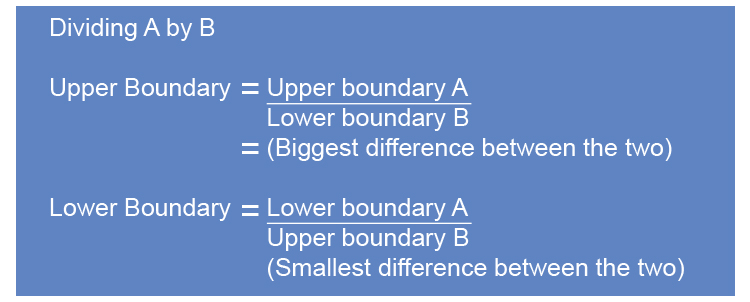# Upper and lower boundary division

If you are looking to divide one quantity by another and you need to know the possible limits of the upper boundary and lower boundary use logic.NOTE:

1.   Upper\ boundary=(Upper\ boundary\ A)/(Lower\ boundary\ B)

If you have the largest possible amount and divide it by the smallest possible amount this will provide you with the biggest divisible number possible.

Upper\ boundary=(Big)/(Small)=Lar\g\e\st\ n\u\mber

2.   Lower\ boundary=(Lower\ boundary\ A)/(Upper\ boundary\ B)

If you have the smallest possible amount and divide it by the largest possible amount this will provide you with the smallest divisible number possible.

Lower\ boundary=(Small)/(Big)=Smal\l\e\st\ n\u\mber

Example 1

3.3m and 4.2m have been rounded to one decimal point.

What are the maximum and minimum values of 3.3div4.2m?

First find the upper and lower boundary of 3.3m which would be:

3.3m to one decimal place.

3.ul3 underline the digit (1^(st) decimal place).

3.ul30 look next door

0      5 or more raises the score. So 3.25 would raise to 3.3.

0       four or less just ignore 3.349dot9.... it would ignore and stay at 3.3.

This is simplified to 3.35

So the upper and lower boundary of 3.3m to 1 D.P. is 3.35m and 3.25m.

The upper and lower boundary of4.2m which would be:

4.2m to one decimal place.

4.ul2 underline the digit (1^(st) decimal place).

4.ul20 look next door

0      5 or more raises the score. So 4.15 would raise to 4.2.

0       four or less just ignore 4.249dot9.... it would ignore and stay at 4.2.

This is simplified to 4.25

So the upper and lower boundary of 4.2m to 1 D.P. is 4.25m and 4.15m.

Summary

Upper and lower boundary of 3.3m is 3.35m and 3.25m

Upper and lower boundary of 4.2m is 4.25m and 4.15m

To work out the upper boundary in division, we use the biggest difference between the two.

So the upper boundary of (3.3)/(4.2) is (Big)/(Small)=(3.35)/(4.15)=0.81 to 2 D.P.

To work out the lower boundary in division, we use the smallest difference between the two.

So the lower boundary of (3.3)/(4.2) is (Small)/(Big)=(3.25)/(4.25)=0.76 to 2 D.P.

Answer: The minimum and maximum values of 3.3div4.2m is 0.81 and 0.76 to 2 D.P.

Example 2

The average fuel consumed by a car is given by the formula:

fuel\ cons\u\m\ed=((d)\ dist\a\nce\ travel\l\e\d\ (kilometres))/((f)\ fuel\ used\ (litres))

If d=143 correct to 3 significant figures

f=13.2 litres correct to 3 significant figures

Work out the lower boundary and upper boundary of fuel consumed.

First find the upper and lower boundary of 143 which would be:

143 correct to 3 significant figures.

14ul3 underline the digit (3^(rd) significant figure).

14ul3.0 look next door

0      5 or more raises the score. So 142.5 would raise to 143.

0       four or less just ignore 143.49dot9..... it would ignore and stay at 143.

This is simplified to 143.5

So the upper and lower boundary of 143 to 3 significant figures is 143.5 and 142.5.

The upper and lower boundary of 13.2 to 3 significant figures would be:

13.2 to 3 significant figures.

13.ul2 underline the digit (3^(rd) significant figure).

13.ul20 look next door

0      5 or more raises the score. So 13.15 would raise to 13.2.

0       four or less just ignore 13.249dot9...... it would ignore and stay at 13.2.

This is simplified to 13.25

So the upper and lower boundary of 13.2 to 3 significant figures is 13.25 and 13.15.

Summary

Upper and lower boundary of 143 is 143.5m and 142.5m

Upper and lower boundary of 13.2 is 13.25m and 13.15m

i. To work out the upper boundary in division, you use the biggest difference between the two.

(d)/(f)=(Big)/(Small)=(143.5)/(13.15)=10.91\ km//l   to 2 D.P.

ii. To work out the lower boundary in division, you use the smallest difference between the two.

(d)/(f)=(Small)/(Big)=(142.5)/(13.25)=10.75\ km//l   to 2 D.P.

The upper boundary of fuel consumed is  10.91\ km//l to 2 D.P.
The lower boundary of fuel consumed is  0.75\ km//l to 2 D.P.